GATE  >  Test: Determinacy & Indeterminacy -1

# Test: Determinacy & Indeterminacy -1

Test Description

## 10 Questions MCQ Test GATE Civil Engineering (CE) 2023 Mock Test Series | Test: Determinacy & Indeterminacy -1

Test: Determinacy & Indeterminacy -1 for GATE 2023 is part of GATE Civil Engineering (CE) 2023 Mock Test Series preparation. The Test: Determinacy & Indeterminacy -1 questions and answers have been prepared according to the GATE exam syllabus.The Test: Determinacy & Indeterminacy -1 MCQs are made for GATE 2023 Exam. Find important definitions, questions, notes, meanings, examples, exercises, MCQs and online tests for Test: Determinacy & Indeterminacy -1 below.
Solutions of Test: Determinacy & Indeterminacy -1 questions in English are available as part of our GATE Civil Engineering (CE) 2023 Mock Test Series for GATE & Test: Determinacy & Indeterminacy -1 solutions in Hindi for GATE Civil Engineering (CE) 2023 Mock Test Series course. Download more important topics, notes, lectures and mock test series for GATE Exam by signing up for free. Attempt Test: Determinacy & Indeterminacy -1 | 10 questions in 30 minutes | Mock test for GATE preparation | Free important questions MCQ to study GATE Civil Engineering (CE) 2023 Mock Test Series for GATE Exam | Download free PDF with solutions
 1 Crore+ students have signed up on EduRev. Have you?
Test: Determinacy & Indeterminacy -1 - Question 1

### Total degree of indeterminacy (both internal and external) of the plane frame shown in the given figure is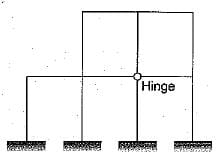Detailed Solution for Test: Determinacy & Indeterminacy -1 - Question 1

The degree of indeterminacy,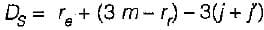Number of external reactions = re
= 3 + 3 + 3 + 3 = 12
Number of rigid joints,
j= 10
Number of joints at which releases are located,
j= 1
Number of members,
m = 12
As the hinge is located at a point where 4 members meet. Hence it is equivalent to three hinges.
Therefore number of releases, r= 3.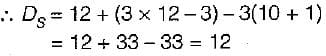Test: Determinacy & Indeterminacy -1 - Question 2

### If there are m unknown member forces, r unknown reaction components and j number of joints, then the degree of static indeterminacy of a pin-jointed plane frame is given by​

Detailed Solution for Test: Determinacy & Indeterminacy -1 - Question 2

Ds for a pin jointed frame = m+r-2j
Ds for a rigid jointed frame = 3m+r -3j
m- No. of members, r- no. of reactions, j- no. of joints

Test: Determinacy & Indeterminacy -1 - Question 3

### Degree of kinematic indeterminacy of a pin jointed plane frame is given by where j is number of joints and r is reaction components.

Detailed Solution for Test: Determinacy & Indeterminacy -1 - Question 3

Dk = 2j –r for a pin jointed frame
Dk = 3j –r for a rigid jointed frame
j number of joints and r number of reactions.

Test: Determinacy & Indeterminacy -1 - Question 4

A pin-jointed plane frame is unstable if where m is number of members r is reaction components and j is number of joints

Test: Determinacy & Indeterminacy -1 - Question 5

The degree of static indeterminacy of a pin- jointed space frame is given by

Test: Determinacy & Indeterminacy -1 - Question 6

The degree of kinematic indeterminacy of a pin-jointed space frame is given by

Test: Determinacy & Indeterminacy -1 - Question 7

The degree of static indeterminacy of the pin- jointed plane frame shown in figure is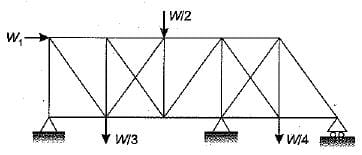Detailed Solution for Test: Determinacy & Indeterminacy -1 - Question 7

External indeterminacy,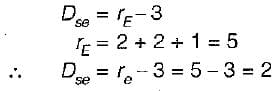Internal indeterminacy,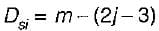No, of members, m = 21
Number of joints, j = 11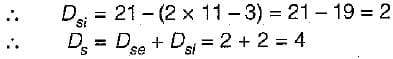Test: Determinacy & Indeterminacy -1 - Question 8

The portal frame as shown in the given frame is statically indeterminate to the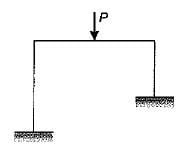Detailed Solution for Test: Determinacy & Indeterminacy -1 - Question 8

m=3; r= 3+3 =6 ; j=4
Ds = 3m+r-3j = 3(3) + 6 -3(4) = 3

Test: Determinacy & Indeterminacy -1 - Question 9

A perfect plane frame having n number of members and j number of joints should satisfy the relation​

Detailed Solution for Test: Determinacy & Indeterminacy -1 - Question 9

A perfect plane frame means a determinate structure, so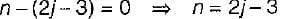Test: Determinacy & Indeterminacy -1 - Question 10

Neglecting axial deformation, the kinematic indeterminacy of the structure shown in the figure below is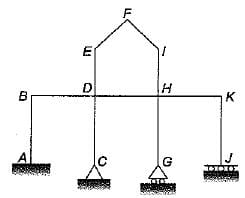Detailed Solution for Test: Determinacy & Indeterminacy -1 - Question 10

j = 11; r=8; m=11
Dk = 3j-r-no. of inextensible members = 3(11) – 8 -11 = 14

## GATE Civil Engineering (CE) 2023 Mock Test Series

26 docs|292 tests
 Use Code STAYHOME200 and get INR 200 additional OFF Use Coupon Code
Information about Test: Determinacy & Indeterminacy -1 Page
In this test you can find the Exam questions for Test: Determinacy & Indeterminacy -1 solved & explained in the simplest way possible. Besides giving Questions and answers for Test: Determinacy & Indeterminacy -1, EduRev gives you an ample number of Online tests for practice

## GATE Civil Engineering (CE) 2023 Mock Test Series

26 docs|292 tests